## 3. 4. 3 Laplace Operator

In the special case of the Laplace operator the vector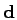has the following form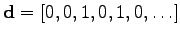(3.60)

For a grid with a regular distance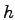between neighboring vertices, the geometrical coefficient matrix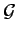yields for five and nine neighboring points: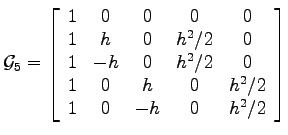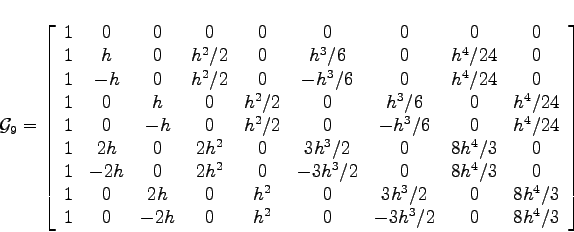After eliminating derivatives which do not appear in the series expansion of the single points, the matrices can be re-written. The derivative vector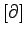is written as: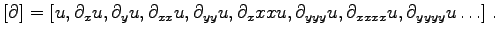(3.61)

The vectoris reduced to the following form: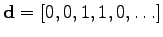(3.62)

Inserting into the formula (3.58) yields the well known expressions. The function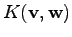can be written as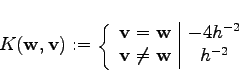For nine points the following formula is obtained: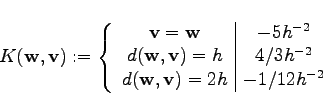Michael 2008-01-16